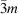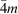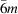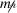International
Tables for
Crystallography
Volume C
Mathematical, physical and chemical tables
Edited by E. Prince

International Tables for Crystallography (2006). Vol. C, ch. 1.4, pp. 15-19

Section 1.4.1. Arithmetic crystal classes

A. J. C. Wilsona

aSt John's College, Cambridge CB2 1TP, England

1.4.1. Arithmetic crystal classes

| top | pdf |

Arithmetic crystal classes are of great importance in theoretical crystallography, and are treated from that point of view in Volume A of International Tables for Crystallography (2005), Section 8.2.3. They have, however, at least four applications in practical crystallography:

 (1) in the classification of space groups (Section 1.4.2); (2) in forming symbols for certain space groups in higher dimensions (see Chapter 9.8and the references cited therein); (3) in modelling the frequency of occurrence of space groups (see Chapter 9.7and the references cited therein); and (4) in establishing `equivalent origins' (Wondratschek, 2005, Section 8.2.3).

The tabulation of arithmetic crystal classes in Volume A is incomplete, and the relation of the notation used in complete tabulations found elsewhere (for example, in Brown, Bülow, Neubüser, Wondratschek & Zassenhaus, 1978) to that of International Tables is not immediately obvious. Simple descriptions and complete enumerations of the arithmetic crystal classes in one, two and three dimensions are therefore given here.

1.4.1.1. Arithmetic crystal classes in three dimensions

| top | pdf |

The 32 geometric crystal classes and the 14 Bravais lattices are familiar in three-dimensional crystallography. The three-dimensional arithmetic crystal classes are easily derived in an elementary fashion by enumerating the compatible combinations of geometric crystal class and Bravais lattice; the symbol adopted by the International Union of Crystallography for an arithmetic crystal class is simply the juxtaposition of the symbol for the geometric crystal class and the symbol for the Bravais lattice (de Wolff et al., 1985). For example, in the monoclinic system the geometric crystal classes are 2, m, and 2/m, and the Bravais lattices are monoclinic P and monoclinic C. The six arithmetic crystal classes in the monoclinic system are thus 2P, 2C, mP, mC, 2/mP, and 2/mC. In certain cases (loosely, when the geometric crystal class and the Bravais lattice have unique directions that are not necessarily parallel), the crystal class and the lattice can be combined in two different orientations. The simplest example is the combination of the orthorhombic crystal class1 mm with the end-centred lattice C. The intersection of the mirror planes of the crystal class defines one unique direction, the C centring of the lattice another. If these directions are placed parallel to one another, the arithmetic class mm2C is obtained; if they are placed perpendicular to one another, a different arithmetic class2 2mmC is obtained. The other combinations exhibiting this phenomenon are lattice P with geometric classes 32, 3m,,, and. By consideration of all possible combinations of geometric class and lattice, one obtains the 73 arithmetic classes listed in Table 1.4.2.1.

1.4.1.2. Arithmetic crystal classes in one, two and higher dimensions

| top | pdf |

In one dimension, there are two geometric crystal classes, 1 and m, and a single Bravais lattice,. Two arithmetic crystal classes result,and. In two dimensions, there are ten geometric crystal classes, and two Bravais lattices, p and c; 13 arithmetic crystal classes result. The two-dimensional geometric and arithmetic crystal classes are listed in Table 1.4.1.1.

 Table 1.4.1.1| top | pdf | The two-dimensional arithmetic crystal classes
Crystal systemCrystal classSpace group
GeometricArithmetic
NumberSymbolNumberSymbol
Oblique 1 1 1p 1 p1
2 2 2p 2 p2
Rectangular m 3 mp 3 pm
4 pg
4 mc 5 cm
2mm 5 2mmp 6 p2mm
7 p2mg
8 p2gg
6 2mmc 9 c2mm
Square 4 7 4p 10 p4
4mm 8 4mmp 11 p4mm
12 p4gm
Hexagonal 3 9 3p 13 p3
3m 10 3m1p 14 p3m1
11 31mp 15 p31m
6 12 6p 16 p6
6mm 13 6mmp 17 p6mm

The number of arithmetic crystal classes increases rapidly with increasing dimensionality; there are 710 (plus 70 enantiomorphs) in four dimensions (Brown, Bülow, Neubüser, Wondratschek & Zassenhaus, 1978), but those in dimensions higher than three are not needed in this volume.

References

Brown, H., Bülow, R., Neubüser, J., Wondratschek, H. & Zassenhaus, H. (1978). Crystallographic groups of four-dimensional space. New York: Wiley.
International Tables for Crystallography (2005). Vol. A. Space-group symmetry, fifth ed., edited by Th. Hahn. Heidelberg: Springer.
Wolff, P. M. de, Belov, N. V., Bertaut, E. F., Buerger, M. J., Donnay, J. D. H., Fischer, W., Hahn, Th., Koptsik, V. A., Mackay, A. L., Wondratschek, H., Wilson, A. J. C. & Abrahams, S. C. (1985). Nomenclature for crystal families, Bravais-lattice types and arithmetic classes. Acta Cryst. A41, 278–280.
Wondratschek, H. (2005). Introduction to space-group symmetry. International tables for crystallography, Vol. A, edited by Th. Hahn, Part 8. Heidelberg: Springer.